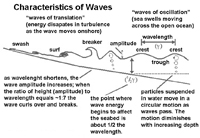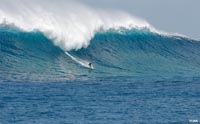# 10.3: Wave Orbits and Orbital Depth

•• Contributed by Miracosta Oceanography 101
• Sourced from Miracosta)

## Wave Orbits and Orbital Depth

Passing waves create a circular current in the water. This is revealed by the orbit-like motion of particles in the water. The orbital motion of a wave is greatest at the surface and diminishes with depth. Orbital depth is the depth to which the orbital motion of the wave energy can be felt. Orbital depth is equal to half of the wavelength. At the sea surface, orbital diameter is equal to wave height. As depth increases, less wave energy can be felt. The orbital depth is the depth where zero wave energy remains. For example, if a wave at the surface has a height of 4 meters and a wavelength of 48 m, then the depth where no motion from the wave exists is 48/2 or 24 meters.Figure 10.6. Orbital oscillations in deep and shallow waves.

## Deep-Water Waves and Shallow-Water Waves

The depth of the water determines the character of wave behaviors.

• Deep-water waves are waves passing through water greater than half of its wavelength. Deep-water waves are waves of oscillation. A wave of oscillation is a wave in the open ocean where movement in the water below a passing wave is in a vertical circular motion.
• Shallow-water waves are waves that are interacting with the seabed in depths less than half it wavelength. Shallow-water waves are called waves of transition because they change character as the move shoreward and dissipate their energy interacting with the seabed onto the shore.Figure 10.7. Waves of oscillation, breakers, and waves of transition moving onto the beach.

 Type of wave Define Orbit Speed Deep water wave (wave of oscillation) d > L/2 Circle L/T Shallow water wave (wave of transition) d < L/20 Elliptical √gd Where... • L/2 to L/20 is a transitional wave • Speed of a shallow water wave is dependent on water depth • G = gravitational constant 9.8 m/s2

Wind waves change as they approach the shore:

• As a wave approaches shallow water its begins to transform when it's orbital depth comes in contact with the seabed (when d < L/2).
• The friction caused by waves interacting with the seabed causes waves to slow down as the move onshore.
• The friction of the seabed begins to slow the bottom of the wave; whereas the top of the wave does not slow as quickly..
• Circular motion within the wave becomes interrupted and becomes elliptical.
• As waves approach the beach, their wavelengths (L) and velocity decrease. However the period (T) stays the same. The shortening of the wavelength results in an increase in wave height as it moves into shallow water.
• A wave breaks when the water depth (d) is about the same as the wave height (h). Where a wave curls over on itself is called a breaker.
• Breakers then turn into a turbulent front called surf that moves onto the beach.
• When the dying wave runs up on the beach and then retreats it is called swash.Figure 10.8. Waves of transition build up, break, and become surf before ending on the beach as swash. Figure 10.9. A breaking wave (with surfer in Puerto Rico)